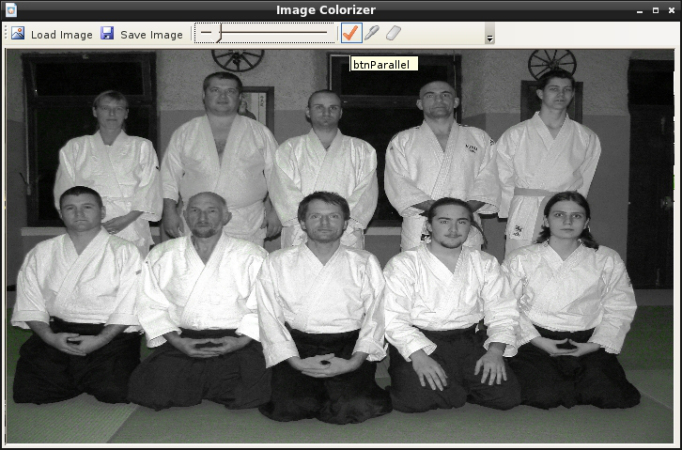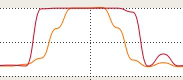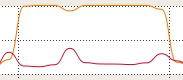This weekend I played with the Microsoft ParallelFx CTP's samples, adapting and running them on current Mono ParallelFx implementation. I'm going to show you two of them and the results. I used the same computer than previously for running the samples.

### Image Colorizer

The first one is an image colorizer. For the test, I used a .jpg file weighting 8,3 Mio and containing 13273085 pixels (4207x3155). The result of the processing is below :For the same color region, the parallel-enabled processing was 1.77x faster than the synchronous one (parallel time : 4.3368363s, non-parallel time : 7.6746839s).

As can be seen on the two following cpu graphs, the parallel version take advantages of both core in my CPU :(Parallel version running)(Non-parallel version running)

### Benchmark sample

The second sample is a benchmark program containing a bunch of test. Each test uses one of the available API in ParallelFx. For the run, I enabled the 3 following benchmarks :

• Matrix multiplication : two random-filled matrix are multiplied together. Both are 500 by 500 matrix.
• Tree sum : all nodes of a binary-tree are added together. I set tree depth to 24 and each node's value was randomly chosen.
• Searching : a random word is searched among all files present in a directory. I used a novel with each chapter split in a distinct .html file. The content of the directory was duplicated so that its total size was 12,6 Mio.

Each of these tests were run 7 times by the program and the time given back was the average of each run with the two extreme values being removed. Following are the results with, in parentheses, the ParallelFx API used by the test.

• Matrix multiplication : 2,25x speedup (Parallel.For).
• Tree sum : 1,58x speedup (Future).
• Searching : 1,37x speedup (Parallel.ForEach).

### Conclusion

So we can see that ParallelFx always provide a boost but that the overall improvement seems to depend on the component used and how it is used. It would be interesting to compare these results with the ones yielded by Microsoft implementation and by the Mono implementation but running on .NET. I will probably do that at the end of GSoC as a conclusion of the work put during the summer ;) .

In addition these samples showed some bugs. Indeed, I had to disable two tests in the benchmark sample because one of them returned different results between parallel/non-parallel version and the other was causing a stack overflow in Mono runtime (I still need to find out if it's Mono fault or mine).